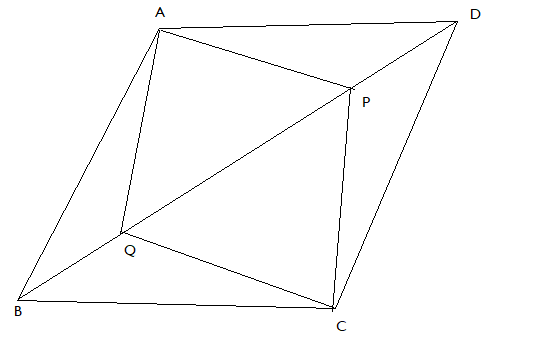QUESTION

# In parallelogram ABCD, two points P and Q are taken on diagonal BD such that DP = BQ. Show that AP = CQ.Hint: We will use congruence to make triangle APD and triangle BQC congruent and by c.p.c.t AP = CQ.

Now, in parallelogram, opposite sides are equal and parallel to each other. Also, the opposite angles are equal in a parallelogram.So, in parallelogram ABCD, AD = BC. Also, AD is parallel to BC and diagonal BD acts as a transversal so, $\angle {\text{ADP = }}\angle {\text{CBQ}}$

Now, in triangle ADP and triangle CBQ,
$\angle {\text{ADP = }}\angle {\text{CBQ}}$ (Alternate interior angles)
So, $\Delta {\text{ADP }} \cong {\text{ }}\Delta {\text{CBQ}}$ By SAS property